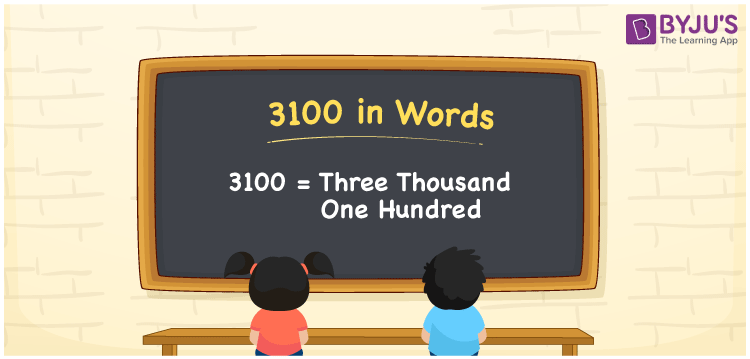# 3100 in Words

3100 in words is written as Three thousand one hundred. In both the International System of Numerals and the Indian System of Numerals, 3100 is written as Three thousand one hundred. The number 3100 is a Cardinal Number as it represents some quantity. For example, “the dress costs 3100 rupees”.

 3100 in words Three thousand one hundred Three thousand one hundred in Number 3100

## 3100 in English Words

3100 in English words is read as “Three thousand one hundred.”## How to Write 3100 in Words?

To write 3100 in words, we shall use the place value chart. In the place value chart, put 3 in the thousands, 1 in the hundreds, and 0 in the tens and the ones place respectively. Let us make a place value chart to write the number 3100 in words.

 Thousands Hundreds Tens Ones 3 1 0 0

Thus, we can write the expanded form as

3 × Thousand + 1 × Hundred + 0 × Ten + 0 × One

= 3 × 1000 + 1 × 100 + 0 × 10 + 0 × 1

= 3000 + 100 + 0 + 0

= 3100

= Three thousand one hundred.

3100 is the natural number that is succeeded by 3099 and preceded by 3101.

3100 in words – Three thousand one hundred

• Is 3100 an odd number? – No
• Is 3100 an even number? – Yes
• Is 3100 a perfect square number? – No
• Is 3100 a perfect cube number? – No
• Is 3100 a prime number? – No
• Is 3100 a composite number? – Yes

## Frequently Asked Questions on 3100 in Words

Q1

### How to write 3100 in words?

3100 in words is written as Three thousand one hundred.
Q2

### How to write 3100 in words in the International and Indian System of Numerals?

In both, the system of numerals, 3100 in words, is written as Three thousand one hundred.
Q3

### How to write 3100 in a place value chart?

In the place value chart, write 3 in the thousands, 1 in the hundreds, and 0 in the tens and the ones, respectively.